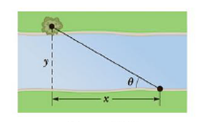# A surveyor measures the distance across a straight river by the following method: starting directly across from a tree on the opposite bank, he walks x = 1.00 × 10 2 m along the riverbank to establish a baseline. Then he sights across to the tree. The angle from his baseline lo the tree is θ = 35.0° (Fig. P1.53). How wide is the river? Figure P1.53### College Physics

11th Edition
Raymond A. Serway + 1 other
Publisher: Cengage Learning
ISBN: 9781305952300### College Physics

11th Edition
Raymond A. Serway + 1 other
Publisher: Cengage Learning
ISBN: 9781305952300

#### Solutions

Chapter
Section
Chapter 1, Problem 53P
Textbook Problem
964 views

## A surveyor measures the distance across a straight river by the following method: starting directly across from a tree on the opposite bank, he walks x = 1.00 × 102 m along the riverbank to establish a baseline. Then he sights across to the tree. The angle from his baseline lo the tree is θ = 35.0° (Fig. P1.53). How wide is the river?Figure P1.53

Expert Solution
To determine
The width of the river.

### Explanation of Solution

Given Info: The surveyor walked distance (x) is about 1×102m . The angle (θ) from the baseline to the tree is 35°

Explanation:

The diagram for the river and the tree is

From the diagram, formula to calculate the width of the river is,

tanθ=wx

• θ is angle,
• w is width of the river,
• x is the walked distance

Substitute 35° for θ , 1×102m for x to find w

### Want to see the full answer?

Check out a sample textbook solution.See solution

### Want to see this answer and more?

Bartleby provides explanations to thousands of textbook problems written by our experts, many with advanced degrees!

See solution